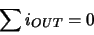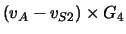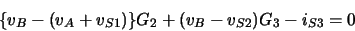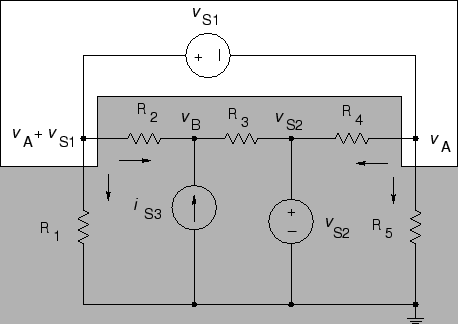Node Current Analysis

1.
Select a reference node. Label each node except the reference with a letter, except:
(a)
If one end of a voltage source is connected to ground, label the other end of the voltage source with the actual voltage (vS2 below).
(b)
If there is a voltage source not connected to ground, label the - end with a letter (vA below), and label the + end with the voltage at the - end plus the voltage of the source ( vA + vS1 below).
2.
For each node except the reference node and nodes connected to voltage sources write KCL in the formwhere iOUT is written in terms of node voltages (e.g.,for R4 below).

The nodal equation for node B below isor

-vA G2 + vB (G2 + G3) = iS3 + vS1 G2 + vS2 G3

(a)
For nodes of the form of 1(a) there is no need to write KCL since the voltage is already known.
(b)
For nodes of the form 1(b) write one KCL for both ends of the voltage source. For example:

The nodal equation for vS1 is

vA G5 + (vA - vS2)G4 + (vA + vS1) G1 + (vA + vS1 - vB) G2 = 0

or

vA (G1 + G2 + G4 + G5) - vB G2 = vS2 G4 - vS1 (G1 + G2)

3.
Solve the equations.